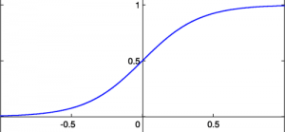# A Brief Introduction to Artificial Neural Networks

31Jan - by Alan - 1 -A Simple Neural Network

This short article gives you a high-level overview of the AI technique known as artificial neural networks (ANN). The objective is to convey intuition rather than rigour, sufficient for example to understand this Python code. After reading this, you might like to follow up with the Further Reading list below. I have made heavy use of Wikipedia (and the listed resources) but any errors are likely my own. There is a companion article over on Python3.codes: A Neural Network in Python, Part 1: sigmoid function, gradient descent & backpropagation

Artificial Neural Networks were first conceived of in the 1940s, but they were of only theoretical interest until some 3 decades later, when the invention of the backpropagation algorithm began to open up the possibilities for practical implementation in software. Along with tremendous advances in computer processing power, and powerful numerical software libraries, its now possible to write useful programs to solve a wide variety of tasks, like computer vision and speech recognition.Biological Neuron

A biological neuron is an electrically excitable cell that processes and transmits information through electrical and chemical signals. These signals between neurons occur via synapses, specialized connections with other cells. Neurons can connect to each other to form neural networks. Dendrites are hair-like extensions of the soma which act like input channels. These input channels receive their input through the synapses of other neurons. The soma processes these incoming signals and then sends that processed value into an output which is sent out to other neurons through the axon and the synapses.By Chrislb (created by Chrislb) [GFDL (http://www.gnu.org/copyleft/fdl.html) or CC-BY-SA-3.0 (http://creativecommons.org/licenses/by-sa/3.0/)], via Wikimedia CommonsAn artificial neural network in AI is a program, or programming paradigm, inspired by the way the brain appears to work, at a low level.

Each neuron receives a number of inputs. Each input’s value is multiplied by a weighting factor for the input channel, then all of the inputs * weights are summed. The initial weights are chosen at random.Sigmoid Function

This sum passes into an activation function which determines the ‘firing value’ of the neuron. A typical activation function is the sigmoid function. A sigmoid function is a mathematical function having an “S” shaped curve (sigmoid curve). Often, sigmoid function refers to the special case of the logistic function defined by the formula S(x) = 1/(1 + ex). There may be a threshold value which the sum must exceed for the neuron to fire.Neural Network Layers

Neurons are grouped into layers, so that there is an input layer, an output layer, and in between are 1 or more ‘hidden’ layers. The input layer is fully connected (each neuron’s output goes to all the neurons of the next layer) to the first hidden layer, which is then fully connected to the next layer, and so on.

At the final layer (the output layer) these ‘guesses’ are compared to the expected results for each given input. Of course, these initial guesses are likely to be terribly wrong! So we compute the sizes of the errors, and the directions that the weights need to be adjusted and backpropagate this information to the previous layers so that they can tweak the weights. This process is called gradient descent. Then we run the forward phase again. This is repeated several times, maybe even thousands or millions of times, and the guesses improve.

Gradient descent utilises a loss function (sometimes referred to as the cost function or error function), which maps values of one or more variables onto a real number intuitively representing some “cost” associated with the weight vector. It calculates the difference between the input training example and its expected output, after the example has been propagated through the network.Gradient Descent

The output of a neuron depends on the weighted sum of all its inputs:, whereandare the weights on the connection from the input units to the output unit (in the case where the neuron has 2 inputs). Therefore, the error also depends on the incoming weights to the neuron, which is ultimately what needs to be changed in the network to enable learning.By AI456 (Graphed with MatLab) [GFDL (http://www.gnu.org/copyleft/fdl.html) or CC BY-SA 3.0 (http://creativecommons.org/licenses/by-sa/3.0)], via Wikimedia CommonsConsider an error (or loss or cost)  functionmeasuring the difference between two outputs. The standard choice is, the square of the Euclidean distance between the vectorsand. The error plots therefore take a parabolic shape. The factor ofconveniently cancels the exponent when the error function is subsequently differentiated. The partial derivative with respect to the outputs is:If each weight is plotted on a separate horizontal axis and the error on the vertical axis, the result is a parabolic bowl. For a neuron withweights, the same plot would require an elliptic paraboloid ofdimensions. The backpropagation algorithm aims to find the set of weights that minimizes the error.

In pseudocode:

  initialize network weights (often small random values)
do
forEach training example named ex
prediction = neural-net-output(network, ex)
actual = teacher-output(ex)
compute error (prediction - actual) at the output
computefor all weights from hidden to output
computefor all weights from input to hidden
update network weights
until stopping criterion satisfied
return the network


This structure can be shown to be capable of learning, such as performing fundamental logical operations, performing simulations of mathematical functions, playing games, recognising handwriting, speech, items in pictures, and so on. The field has advanced incredibly in recent years, such that these programs can match or even surpass human experts. This is in contrast to ‘algorithmic’ programming, which depends on explicitly specifying the steps required to solve a problem. This can easily become too complex and unwieldy for practical purposes, but an ANN operates more like humans by learning from examples – lots of them.

## One thought on “A Brief Introduction to Artificial Neural Networks”

This site uses Akismet to reduce spam. Learn how your comment data is processed.